# Field-effect transistors

Home - Techniek - Electronica - Radiotechniek - Radio amateur bladen - QST - Field-effect transistors

What they are - How they work.

A recently introduced semiconductor device called the field-effect transistor, or FET for short, combines some of the best features of both the vacuum tube and the transistor. The FET is appearing in new electronic circuits of all types and will soon be used in amateur radio equipment. A great deal of excitement centers about this solid-state device which acts much like a vacuum tube, and it is the purpose of this article to describe its construction and operation.

Before getting into device characteristics, let us quickly review some basic concepts in semiconductors. Useful semiconductor material is either p-type, where holes (a hole is actually a place where there is no electron) are concentrated relatively heavily, or n-type which features an excess of free electrons. Both the holes and free electrons are the current carriers in a piece of semiconductor, just as free electrons only are the current carriers in a piece of copper. As a memory aid, remember that the "p" in p-type material stands for the positive charge of the current carriers (holes) and the "n" stands for the negative charge of the current carriers (free electrons) in n-type semiconductor material. When p-type material and n-type material are brought together, a p-n junction is formed as shown in Fig. 1A.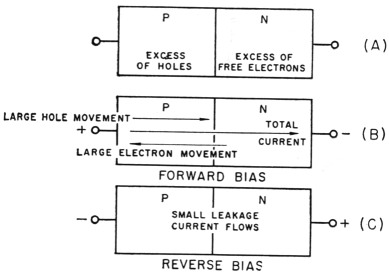Fig. 1. Action of p-n junction when bias is applied.

### The junction diode

The resulting action of a p-n junction is well known. When a forward bias is applied as shown in Fig. 1B, a large current will flow. The total current is made up of the electron current plus the hole current. When reverse bias (Fig. 1C) is applied, only a small reverse current flows - the p-n junction acts as a diode. It is important to note the reverse-bias case. We know that when a voltage is applied to a resistive network, this voltage is dissipated by IR drops until the applied voltage is "used up" by the sum total of all the voltage drops. The same principle applies to the case of the reverse-biased p-n junction diode. We have applied V volts of reverse bias and this voltage must be used up somehow in our circuit, the p-n junction. The resulting action is shown in Fig. 2, where charges are separated in a region close to the junction. Enough charges are moved until the electric field across the junction produces a voltage drop which is approximately equal to our applied voltage. The important point here is that the region around the p-n junction is now depleted of all its holes and free electrons, thus there are no current carriers available. This region is commonly called the "depletion region" and it sounds reasonable that its thickness depends on the magnitude of reverse voltage which is applied. It will be an important point in the operation of field-effect transistors that no current can flow in the depletion region since there are no current carriers in that region.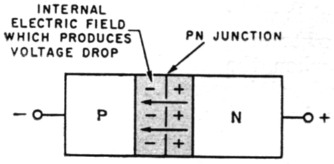Fig. 2. Reverse bias causes electrons and holes to move away from the p-n junction, leaving a depletion region (shaded area) and no current flows.

The field-effect transistor, for the last few years a tantalizing promise of better things in semiconductor devices, is now rapidly coming into the market at prices attractive to amateurs. We've already shown you one application in Walt Lange's audio oscillator (July QST). Here's the background story of the FET - how it's made and how it functions, and why it will be superior to older transistor types in many applications.

### The junction FET

Field-effect transistors are divided into two main groups: junction FETs, and insulated-gate FETs. We will discuss the Junction FET, or JFET, first. The basic JFET device is shown in Fig. 3.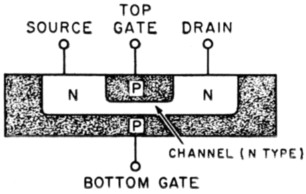Fig. 3. The junction field-effect transistor.

First of all, note the location of the terminals where voltages can be applied. The reason for the terminal names will become clear later. A d.c. operating condition is set up by starting a current flow between source and drain. This current flow is made up of free electrons since the semiconductor is n-type in the channel, so a positive voltage is applied at the drain. This positive voltage attracts the negatively-charged free electrons and the current flows (Fig. 4A). The next step is to apply a gate voltage of the polarity shown in Fig. 4B. Note that this reverse-biases the gates with respect to the source, channel, and drain. This reverse-bias gate voltage causes a depletion layer to be formed which takes up part of the channel, and since the electrons now have less volume in which to move the resistance is greater and the current between source and drain is reduced. If we apply a large gate voltage, we cause the depletion regions to meet, and in this case the source-drain current is reduced nearly to zero. Since we changed the large source-drain current with a relatively small gate-voltage, we have a device which acts as an amplifier.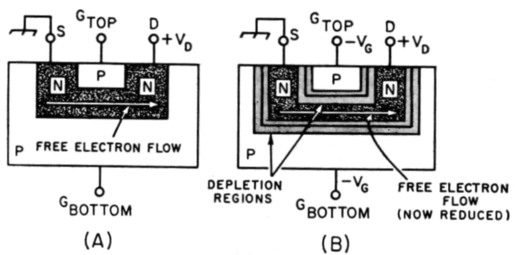Fig. 4. Operation of the JFET under applied bias. A depletion region (light shading) is formed, compressing the channel and increasing its resistance to current flow.

Further, note that in the operation of the JFET, the gate terminal is never forward biased, because if it were the source-drain current would all be diverted through the forward-biased gate junction diode.

The resistance between the gate terminal and the rest of the device is very high, since the gate terminal is always reverse biased, so the JFET has a very high input resistance. The source terminal is the source of current carriers, and they are drained out of the circuit at the drain. The gate opens and doses the amount of channel current which flows. It is seen how the operation of a FET closely resembles the operation of the vacuum tube with its high grid input impedance. Comparing the JFET to a vacuum tube, the source corresponds to the cathode, the gate to the grid, and the drain to the plate.

### Insulated-gate FET

The other large family which makes up field-effect transistors is the insulated-gate field-effect transistor, or IGFET, which is pictured schematically in Fig. 5. In order to set up a d.c. operating condition, a positive polarity is applied to the drain terminal. The substrate is connected to the source, and both are at ground potential, so the channel electrons are attracted to the positive drain and we now have a d.c. source-drain current. In order to regulate this current, we apply voltage to the gate contact. Note that the gate is insulated from the rest of the device by a piece of insulating glass so this is not a p-n junction between the gate and the device - thus the name insulated gate. When a negative gate polarity is applied, positively-charged holes from the p-type substrate are attracted towards the gate and the conducting channel is made more narrow; thus the source-drain current is reduced. When we connect a positive gate voltage, the holes in the substrate are repelled away, the conducting channel is made larger, and the source-drain current is increased. As can be seen, the IGFET is more flexible since we can apply either a positive or negative voltage to the gate. The resistance between the gate and the rest of the device is extremely high because they are separated by a layer of glass-not as clear as your window glass, but it conducts just as poorly. Thus the IGFET has an extremely high input impedance. In fact, since the leakage through the insulating glass is generally much smaller than through the reverse-biased p-n gate junction in the JFET, the IGFET has a much higher input impedance. Typical values of Rin for the IGFET are over a million megohms, while Rin for the JFET ranges from megohms to over a thousand megohms.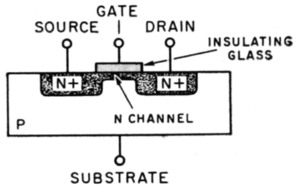Fig. 5. The insulated-gate field-effect transistor.

### Characteristic curves

The characteristic curves for the FETs described above are shown in Figs. 6 and 7, where drain-source current is plotted against drain-source voltage for given values of the gate voltage. Note the similarity to the family of a vacuum-tube pentode as shown in Fig. 8, where plate current is plotted against plate voltage for varying amounts of grid voltage.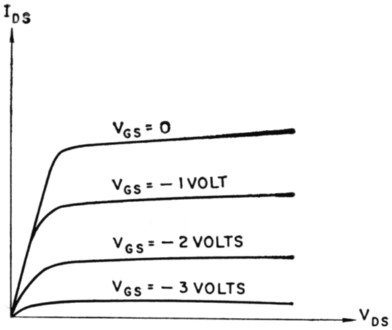Fig. 6. Typical JFET characteristic curves.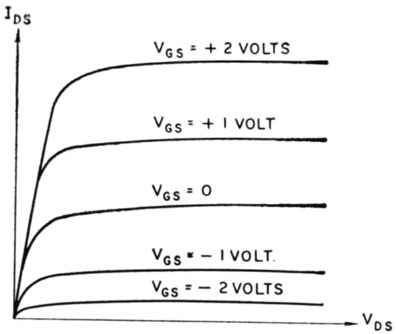Fig. 7. Typical IGFET characteristic curves.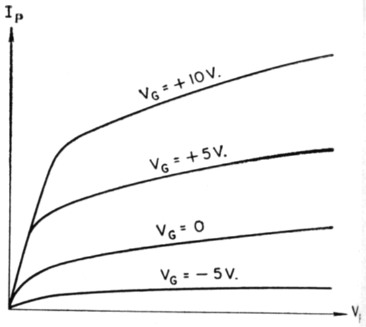Fig. 8-Typical vacuum-tube characteristic curve.

In discussing the JFET so far we have left both gates separate so the device can be used as a tetrode in mixer applications. However, the gates can be internally connected for triode applications. When using the IGFET the substrate is always a.c.-shorted to the source, and only the insulated gate is used to control the current flow. This is done so that both positive and negative polarities can be applied to the device, as opposed to JFET operation where only one polarity can be used, because if the gate itself becomes forward biased the unit is no longer useful.

### Classifications

Field-effect transistors are classed into tact main groupings for application in circuits, enhancement mode and depletion mode. The enhancement-mode devices are those specifically constructed so that they have no channel. They become useful only when we apply a gate voltage which causes a channel to be formed. IGFETs can be used as enhancement-mode devices since both polarities can be applied to the gate without the gate becoming forward biased and conducting current.

A depletion-mode unit corresponds to Figs. 3 and 5 shown earlier, where a channel exists with no gate voltage applied. For the JFET we can apply a gate voltage and deplete the channel, causing the current to decrease. With the IGFET we can apply a gate voltage of either polarity so the device can be depleted (current decreased) or enhanced (current increased).

To sum up, a depletion-mode FET is one which has a channel constructed; thus it has a current flow for zero gate voltage. Enhancement-mode FETs are those which have no channel, so no current flows with zero gate voltage. The latter type devices are especially useful in logic applications.

Circuit symbols approved for FETs are shown in Fig. 9. Both depletion-mode and enhancement-mode devices are illustrated.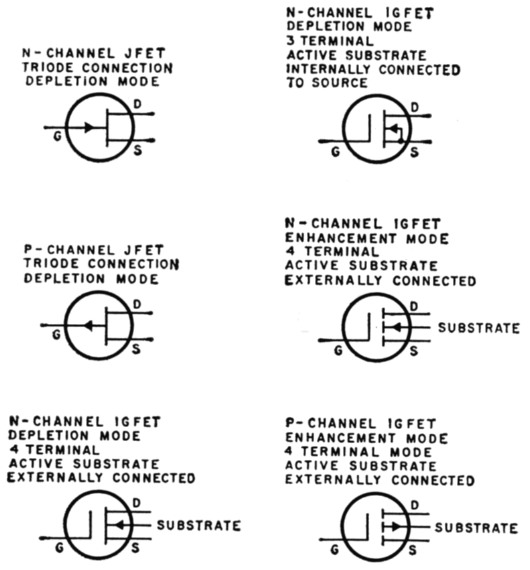Fig. 9. Symbols for most-commonly available field-effect transistors.

### Applications

Some applications for FETs are shown in Figs. 10 and 11. In Fig. 10 a JFET oscillator is pictured, and a versatile FET d.c. voltmeter (FETVM) is shown in Fig. 11.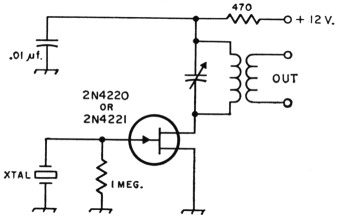Fig. 10. Typical JFET crystal-oscillator circuit.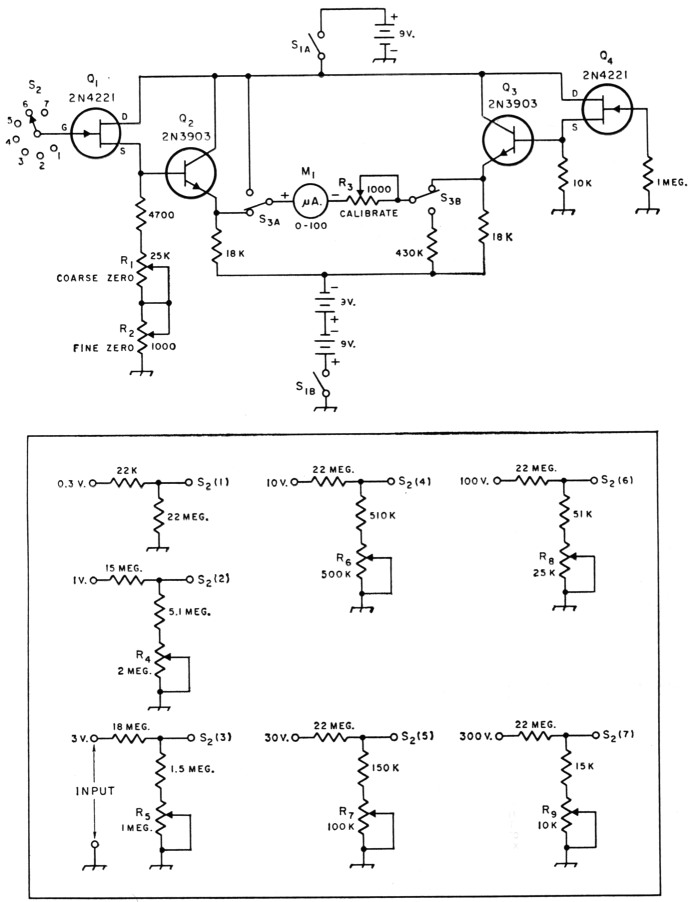Fig. 11. D.C. voltmeter circuit using field-effect transistor. Resistances are in ohms (K = 1000); fixed resistors are 1/2 watt. The second position of Ss is used for checking battery voltage.
M1 0-100 microammeter.
S1 D.p.s.t. toggle.
S2 D.p.d.t. toggle.
Sa Rotary, 1 section, 1 pole, 7 positions.
R1,R2,R3 Linear controls (R2 and Ra are panel adjustments; RI can be internal).
R4-R9 inc.-Trimming potentiometers, for internal mounting (Mallory MTC or equivalent).

The voltmeter features two Motorola 2N4221 JFETs and offers the high input impedance (22 megohms on all ranges in Fig. 11) of a v.t.v.m. but with more stability. The circuit is essentially a differential amplifier which works on the principle that the current through a resistance is directly proportional to the difference between the voltages at its ends. When RI and R2 are adjusted so that the voltage at the emitter of Q2 is equal to the voltage at the emitter of Q3, with no input signal, the voltage difference is zero and no current will flow through the meter.

When the voltage at the gate, G, of Ql is raised to 0.3 volt the voltage across the meter circuit also is approximately 0.3 volt since the source followers and emitter followers have a voltage gain of approximately one. R3 is then adjusted to give full-scale meter deflection. The calibration resistors, R4-82 inclusive, are individually adjusted for exactly full-scale deflection on each range when the maximum voltage for that range is applied to the input terminals through the range switch, 82. An accurate voltmeter should be used to check the applied voltage when setting the calibration resistors.

These two circuits were furnished by Don Wollesen and Walter Birks of the Applications Engineering Group at Motorola Semiconductor Products Division.

This article has provided a basic look into the FET and some of its applications. In general, the FET offers much improved noise performance, stability, and cross-modulation resistance over either vacuum tubes or standard transistors (p-n-p, n-p-n). Its many features, such as high input impedance and desirable high-frequency performance, insure its design into a vast number of electronic circuits in the near future. It is probably the most recent radical improvement, in device design, and should prove most interesting to amateur radio operators everywhere, allowing them greatly improved freedom in circuit design.

Jim George, W7AWH.